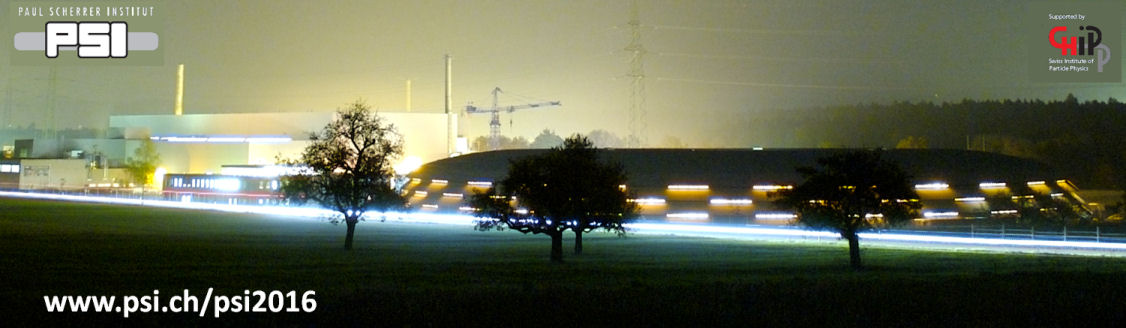#Physics of fundamental Symmetries and Interactions - PSI2016

Oct 16 – 20, 2016
Europe/Zurich timezone

## Cartan's Torsion as Origin for Cosmological Constant and Dark Energy Density

Oct 18, 2016, 6:51 PM
1m
Poster Searches for symmetry violations and new forces

### Speaker

Prof. A.N. Ivanov (TU Wien)

### Description

We analyse the Einstein--Cartan gravity in its standard form ${\cal R} = R + {\cal K}^2$, where ${\cal R}$ and $R$ are the Ricci scalar curvatures in the Einstein--Cartan and Einstein gravity, respectively, and ${\cal K}^2$ is the quadratic contribution of torsion in terms of the contorsion tensor ${\cal K}$. We treat torsion as an external (or a background) field and show that the contribution of torsion to the Einstein equations can be interpreted in terms of the torsion energy--momentum tensor, local conservation of which in a curved spacetime with an arbitrary metric or an arbitrary gravitational field demands a proportionality of the torsion energy--momentum tensor to a metric tensor, a covariant derivative of which vanishes because of the metricity condition. This allows to claim that torsion can serve as origin for vacuum energy density, given by cosmological constant or dark energy density in the Universe. This is a model--independent result may explain a small value of cosmological constant, which is a long--standing problem of cosmology. We show that the obtained result is valid also in the Poincar\'{e} gauge gravitational theory by Kibble (T. W. B. Kibble, J. Math. Phys. {\bf 2}, 212 (1961)), where the Einstein--Hilbert action can be represented in the same form ${\cal R} = R + {\cal K}^2$.

### Primary author

Prof. A.N. Ivanov (TU Wien)

### Co-author

M. Wellenzohn (TU Wien)

### Presentation materials

There are no materials yet.